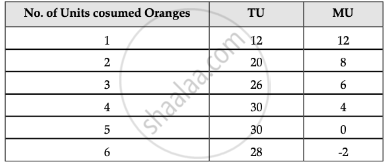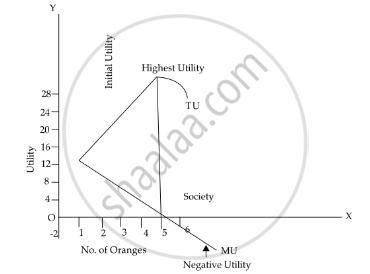# The Law of Diminishing Marginal Utility Can Be Explained with the Help of Schedule and Diagram. - Economics

Diagram
Short Note

The law of diminishing marginal utility can be explained with the help of schedule and diagram.

#### Solution

‘Other things remaining the same, when a person takes successive units of a commodity, the marginal utility diminishes constantly.’

The marginal utility of a commodity diminishes at the consumer gets larger quantities of it. Marginal utility is the change in the total utility resulting from one unit change in the consumption of a commodity per unit of time. Assumptions :
Followings are the some assumptions of the law of diminishing marginal utility :
(i) It is assumed that utility can be measured and a consumer can express his satisfaction in quantitative terms such as 1, 2, 3... etc.
(ii) Consumption of reasonable quantity.
(iii) Continuous consumption.
(iv) Monetary measurement of utility.
(v) No change in quantity.
(vi) Rational consumer.
(vii) MU of money remains constant.
(viii)Independent utilities.
(ix) Fixed income price.
This law can be explained with the help of the above schedule and diagram:Concept: Diminishing Marginal Utility
Is there an error in this question or solution?
2015-2016 (March)

Share